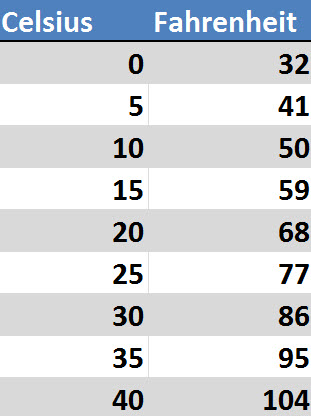# Converting Celsius to Fahrenheit

The United States is one of the few remaining countries using the Fahrenheit scale to measure temperature. Most countries use Celsius. Fortunately, converting Celsius to Fahrenheit is not too difficult. It can be done with some simple math. You can also memorize some of the main temperature conversions or consult a chart. You can also use a search engine.

Celsius has a melting point of zero (0°) and a boiling point of 100°. The concept behind Celsius is that it is easier to use because of this scale. Fahrenheit has a melting point of 32° and a boiling point of 212°. A person could also argue that Fahrenheit is better for weather temperatures because it describes the temperature quickly without the person having to consider decimals.

Mathematical Conversion

To convert from Celsius to Fahrenheit you multiple the Celsius temperature by 1.8 and add 32.

To convert from Fahrenheit to Celsius you substract 32 and then multiply by 5/9 (.5556).

A Lifehacker article notes that you can use 2 as your multiplier instead of 1.8 and then add 32 and get a number that is fairly close to the more exact result.

You can also remember that 20° Celsius is room temperature, 25° Celsius is just getting out of the mid 70s on Fahrenheit, 30° Celsius and its starts getting quite warm outside (above the mid 80s) and 40° degrees Celsius is a very hot summer temperature.

Conversions Chart

Here are some common conversions.
• 10 Celsius° is 50° Fahrenheit
• 20 Celsius° is 68° Fahrenheit
• 25 Celsius° is 77° Fahrenheit
• 30 Celsius° is 86° Fahrenheit
• 37 Celsius° is 98.6° Fahrenheit
• 40 Celsius° is 104° FahrenheitYou can find a bigger list of already convert temperatures on Rapid Tables.